# Thermodynamics chapter 3

8 de Nov de 2016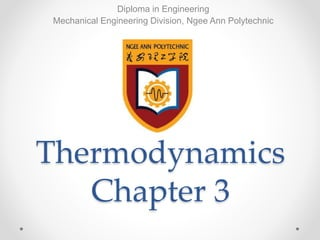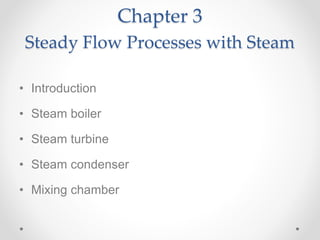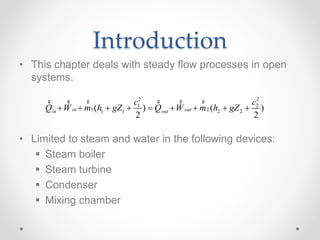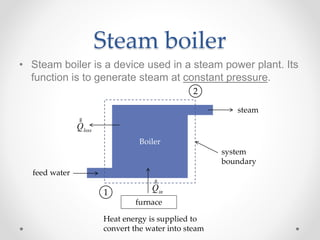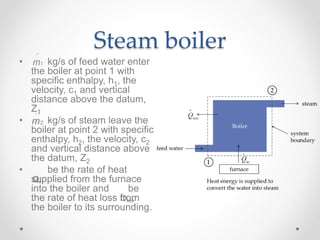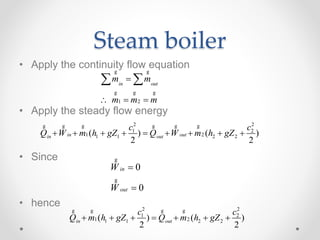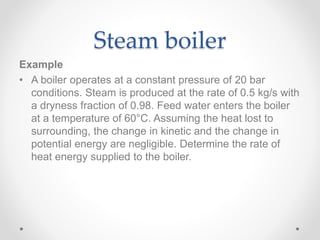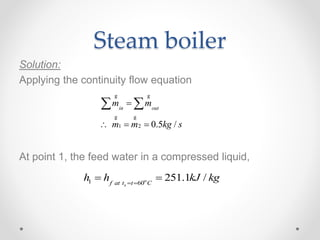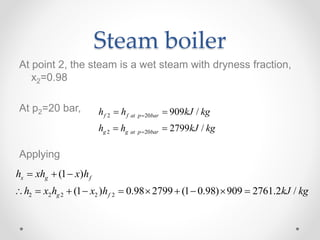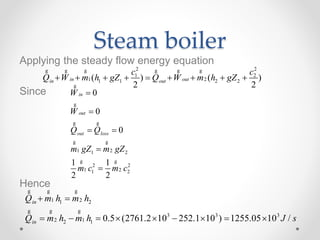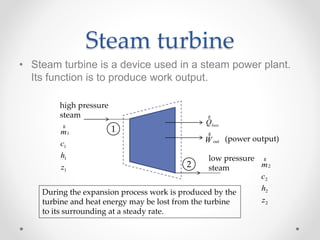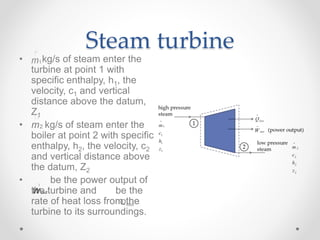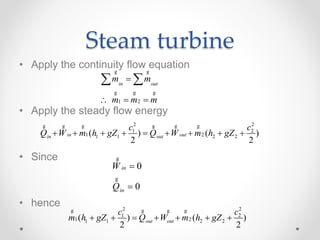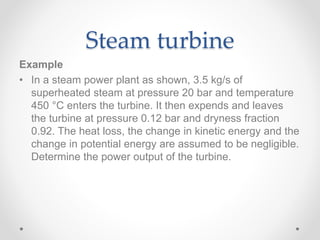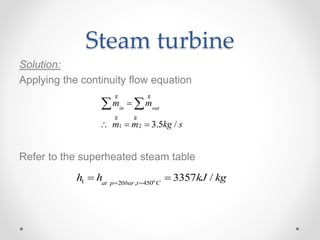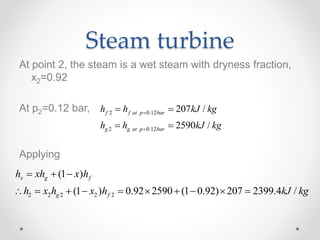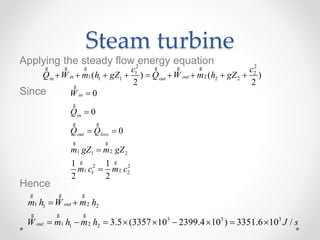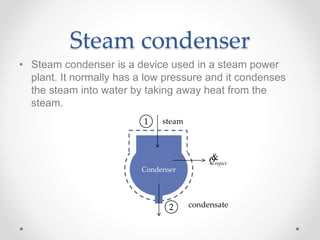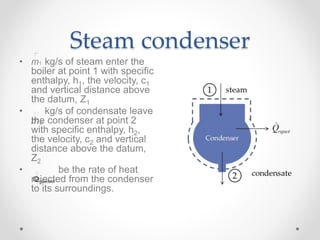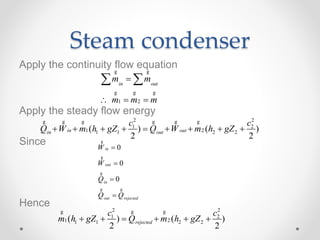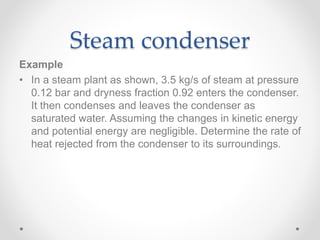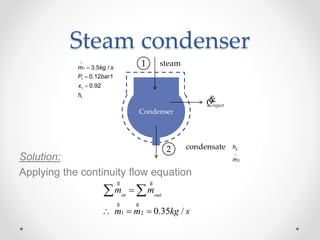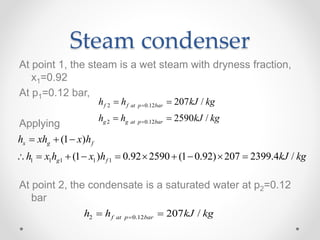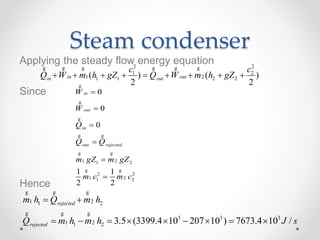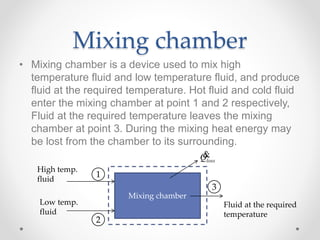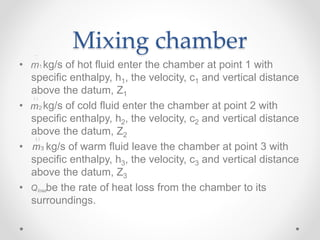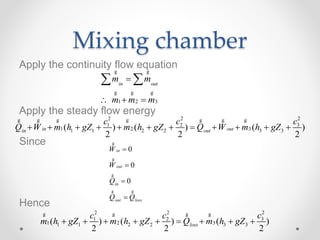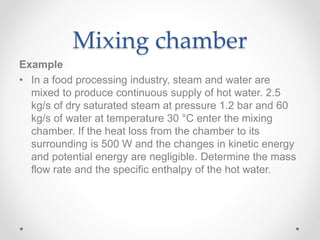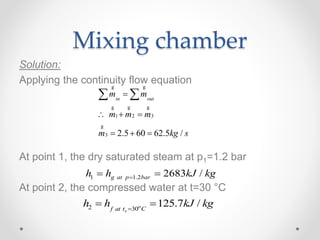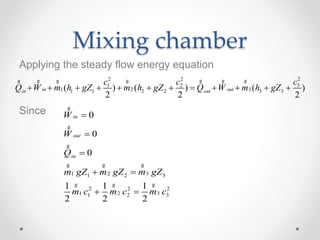1 de 32

### Thermodynamics chapter 3

• 1. Thermodynamics Chapter 3 Diploma in Engineering Mechanical Engineering Division, Ngee Ann Polytechnic
• 2. Chapter 3 Steady Flow Processes with Steam • Introduction • Steam boiler • Steam turbine • Steam condenser • Mixing chamber
• 3. Introduction • This chapter deals with steady flow processes in open systems. • Limited to steam and water in the following devices:  Steam boiler  Steam turbine  Condenser  Mixing chamber 2 2 1 2 1 21 1 2 2( ) ( ) 2 2 in outin out c c Q W m h gZ Q W m h gZ         g g g g g g
• 4. Steam boiler • Steam boiler is a device used in a steam power plant. Its function is to generate steam at constant pressure. Boiler steam feed water furnace inQ g lossQ g system boundary 1 2 Heat energy is supplied to convert the water into steam
• 5. Steam boiler • kg/s of feed water enter the boiler at point 1 with specific enthalpy, h1, the velocity, c1 and vertical distance above the datum, Z1 • kg/s of steam leave the boiler at point 2 with specific enthalpy, h2, the velocity, c2 and vertical distance above the datum, Z2 • be the rate of heat supplied from the furnace into the boiler and be the rate of heat loss from the boiler to its surrounding.
• 6. Steam boiler • Apply the continuity flow equation • Apply the steady flow energy • Since • hence 2 2 1 2 1 21 1 2 2( ) ( ) 2 2 in outin out c c Q W m h gZ Q W m h gZ         g g g g g g 1 2 in out m m m m m       g g g g g 0 0 in out W W   g g 2 2 1 2 1 21 1 2 2( ) ( ) 2 2 in out c c Q m h gZ Q m h gZ       g g g g
• 7. Steam boiler Example • A boiler operates at a constant pressure of 20 bar conditions. Steam is produced at the rate of 0.5 kg/s with a dryness fraction of 0.98. Feed water enters the boiler at a temperature of 60°C. Assuming the heat lost to surrounding, the change in kinetic and the change in potential energy are negligible. Determine the rate of heat energy supplied to the boiler.
• 8. Steam boiler Solution: Applying the continuity flow equation At point 1, the feed water in a compressed liquid, 1 2 0.5 / in out m m m m kg s       g g g g 1 60 251.1 / sf at t t C h h kJ kg   o
• 9. Steam boiler At point 2, the steam is a wet steam with dryness fraction, x2=0.98 At p2=20 bar, Applying 2 20 2 20 909 / 2799 / f f at p bar g g at p bar h h kJ kg h h kJ kg       2 2 2 2 2 (1 ) (1 ) 0.98 2799 (1 0.98) 909 2761.2 / x g f g f h xh x h h x h x h kJ kg             
• 10. Steam boiler Applying the steady flow energy equation Since Hence 2 2 1 2 1 21 1 2 2( ) ( ) 2 2 in outin out c c Q W m h gZ Q W m h gZ         g g g g g g 1 21 2 2 2 1 21 2 0 0 0 1 1 2 2 in out out loss W W Q Q m gZ m gZ m c m c       g g g g g g g g 1 21 2 3 3 3 2 12 1 0.5 (2761.2 10 252.1 10 ) 1255.05 10 / in in Q m h m h Q m h m h J s            g g g g g g
• 11. Steam turbine • Steam turbine is a device used in a steam power plant. Its function is to produce work output. lossQ g outW g (power output) 1 2 high pressure steam low pressure steam 1 1 1 1 m c h z g 2 2 2 2 m c h z g During the expansion process work is produced by the turbine and heat energy may be lost from the turbine to its surrounding at a steady rate.
• 12. Steam turbine • kg/s of steam enter the turbine at point 1 with specific enthalpy, h1, the velocity, c1 and vertical distance above the datum, Z1 • kg/s of steam enter the boiler at point 2 with specific enthalpy, h2, the velocity, c2 and vertical distance above the datum, Z2 • be the power output of the turbine and be the rate of heat loss from the turbine to its surroundings.
• 13. Steam turbine • Apply the continuity flow equation • Apply the steady flow energy • Since • hence 2 2 1 2 1 21 1 2 2( ) ( ) 2 2 in outin out c c Q W m h gZ Q W m h gZ         g g g g g g 1 2 in out m m m m m       g g g g g 0 0 in in W Q   g g 2 2 1 2 1 21 1 2 2( ) ( ) 2 2 outout c c m h gZ Q W m h gZ       g g g g
• 14. Steam turbine Example • In a steam power plant as shown, 3.5 kg/s of superheated steam at pressure 20 bar and temperature 450 °C enters the turbine. It then expends and leaves the turbine at pressure 0.12 bar and dryness fraction 0.92. The heat loss, the change in kinetic energy and the change in potential energy are assumed to be negligible. Determine the power output of the turbine.
• 15. Steam turbine Solution: Applying the continuity flow equation Refer to the superheated steam table 1 2 3.5 / in out m m m m kg s       g g g g 1 20 , 450 3357 /at p bar t C h h kJ kg   o
• 16. Steam turbine At point 2, the steam is a wet steam with dryness fraction, x2=0.92 At p2=0.12 bar, Applying 2 0.12 2 0.12 207 / 2590 / f f at p bar g g at p bar h h kJ kg h h kJ kg       2 2 2 2 2 (1 ) (1 ) 0.92 2590 (1 0.92) 207 2399.4 / x g f g f h xh x h h x h x h kJ kg             
• 17. Steam turbine Applying the steady flow energy equation Since Hence 2 2 1 2 1 21 1 2 2( ) ( ) 2 2 in outin out c c Q W m h gZ Q W m h gZ         g g g g g g 1 21 2 2 2 1 21 2 0 0 0 1 1 2 2 in in out loss W Q Q Q m gZ m gZ m c m c       g g g g g g g g 1 21 2 3 3 3 1 21 2 3.5 (3357 10 2399.4 10 ) 3351.6 10 / out out m h W m h W m h m h J s            g g g g g g
• 18. Steam condenser • Steam condenser is a device used in a steam power plant. It normally has a low pressure and it condenses the steam into water by taking away heat from the steam. Condenser 1 2 steam condensate rejectQ&
• 19. Steam condenser • kg/s of steam enter the boiler at point 1 with specific enthalpy, h1, the velocity, c1 and vertical distance above the datum, Z1 • kg/s of condensate leave the condenser at point 2 with specific enthalpy, h2, the velocity, c2 and vertical distance above the datum, Z2 • be the rate of heat rejected from the condenser to its surroundings.
• 20. Steam condenser Apply the continuity flow equation Apply the steady flow energy Since Hence 2 2 1 2 1 21 1 2 2( ) ( ) 2 2 in outin out c c Q W m h gZ Q W m h gZ         g g g g g g 1 2 in out m m m m m       g g g g g 0 0 0 in out in out rejected W W Q Q Q     g g g g g 2 2 1 2 1 21 1 2 2( ) ( ) 2 2 rejected c c m h gZ Q m h gZ      g g g
• 21. Steam condenser Example • In a steam plant as shown, 3.5 kg/s of steam at pressure 0.12 bar and dryness fraction 0.92 enters the condenser. It then condenses and leaves the condenser as saturated water. Assuming the changes in kinetic energy and potential energy are negligible. Determine the rate of heat rejected from the condenser to its surroundings.
• 22. Steam condenser Solution: Applying the continuity flow equation 1 2 0.35 / in out m m m m kg s       g g g g Condenser 1 2 steam condensate rejectQ& 1 1 1 1 3.5 / 0.12 1 0.92 m kg s P bar x h    2 2 h m
• 23. Steam condenser At point 1, the steam is a wet steam with dryness fraction, x1=0.92 At p1=0.12 bar, Applying At point 2, the condensate is a saturated water at p2=0.12 bar 2 0.12 2 0.12 207 / 2590 / f f at p bar g g at p bar h h kJ kg h h kJ kg       1 1 1 1 1 (1 ) (1 ) 0.92 2590 (1 0.92) 207 2399.4 / x g f g f h xh x h h x h x h kJ kg              2 0.12 207 /f at p barh h kJ kg 
• 24. Steam condenser Applying the steady flow energy equation Since Hence 2 2 1 2 1 21 1 2 2( ) ( ) 2 2 in outin out c c Q W m h gZ Q W m h gZ         g g g g g g 1 21 2 2 2 1 21 2 0 0 0 1 1 2 2 in out in out rejected W W Q Q Q m gZ m gZ m c m c       g g g g g g g g g 1 21 2 3 3 3 1 21 2 3.5 (3399.4 10 207 10 ) 7673.4 10 / rejected rejected m h Q m h Q m h m h J s            g g g g g g
• 25. Mixing chamber • Mixing chamber is a device used to mix high temperature fluid and low temperature fluid, and produce fluid at the required temperature. Hot fluid and cold fluid enter the mixing chamber at point 1 and 2 respectively, Fluid at the required temperature leaves the mixing chamber at point 3. During the mixing heat energy may be lost from the chamber to its surrounding. Mixing chamber 1 2 3 High temp. fluid Low temp. fluid Fluid at the required temperature lossQ&
• 26. Mixing chamber • kg/s of hot fluid enter the chamber at point 1 with specific enthalpy, h1, the velocity, c1 and vertical distance above the datum, Z1 • kg/s of cold fluid enter the chamber at point 2 with specific enthalpy, h2, the velocity, c2 and vertical distance above the datum, Z2 • kg/s of warm fluid leave the chamber at point 3 with specific enthalpy, h3, the velocity, c3 and vertical distance above the datum, Z3 • be the rate of heat loss from the chamber to its surroundings.
• 27. Mixing chamber Apply the continuity flow equation Apply the steady flow energy Since Hence 22 2 31 2 1 2 31 1 2 2 3 3( ) ( ) ( ) 2 2 2 in outin out cc c Q W m h gZ m h gZ Q W m h gZ            g g g g g g g 1 2 3 in out m m m m m       g g g g g 0 0 0 in out in out loss W W Q Q Q     g g g g g 22 2 31 2 1 2 31 1 2 2 3 3( ) ( ) ( ) 2 2 2 loss cc c m h gZ m h gZ Q m h gZ         g g g g
• 28. Mixing chamber Example • In a food processing industry, steam and water are mixed to produce continuous supply of hot water. 2.5 kg/s of dry saturated steam at pressure 1.2 bar and 60 kg/s of water at temperature 30 °C enter the mixing chamber. If the heat loss from the chamber to its surrounding is 500 W and the changes in kinetic energy and potential energy are negligible. Determine the mass flow rate and the specific enthalpy of the hot water.
• 29. Mixing chamber Solution: Applying the continuity flow equation At point 1, the dry saturated steam at p1=1.2 bar At point 2, the compressed water at t=30 °C 1 2 3 3 2.5 60 62.5 / in out m m m m m m kg s          g g g g g g 1 1.2 2683 /g at p barh h kJ kg  2 30 125.7 / sf at t C h h kJ kg  o
• 30. Mixing chamber Applying the steady flow energy equation Since 1 2 31 2 3 2 2 2 1 2 31 2 3 0 0 0 1 1 1 2 2 2 in out in W W Q m gZ m gZ m gZ m c m c m c        g g g g g g g g g 22 2 31 2 1 2 31 1 2 2 3 3( ) ( ) ( ) 2 2 2 in outin out cc c Q W m h gZ m h gZ Q W m h gZ            g g g g g g g
• 31. Mixing chamber Hence 1 2 31 2 3 3 1 23 1 2 1 21 2 3 3 3 3 3 2.5 2683 10 60 125.7 10 500 62.5 227.98 10 / loss loss loss m h m h Q m h m h m h m h Q m h m h Q h m J kg                    g g g g g g g g g g g g